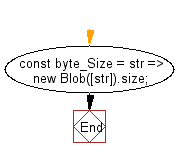# JavaScript: Convert the length of a given string in bytes

## JavaScript fundamental (ES6 Syntax): Exercise-13 with Solution

Write a JavaScript program to convert the length of a given string in bytes.

• Convert a given string to a Blob Object.
• Use Blob.size to get the length of the string in bytes.

Sample Solution:

JavaScript Code:

``````//#Source https://bit.ly/2neWfJ2
const byte_Size = str => new Blob([str]).size;
console.log(byte_Size('123456'));
console.log(byte_Size('Hello World'));
console.log(byte_Size('Ã¢'));
```
```

Sample Output:

```6
11
4
```

Pictorial Presentation:Flowchart:Live Demo:

See the Pen javascript-basic-exercise-1-13 by w3resource (@w3resource) on CodePen.

Improve this sample solution and post your code through Disqus

What is the difficulty level of this exercise?

Test your Programming skills with w3resource's quiz.

﻿

## JavaScript: Tips of the Day

Chunks an array into n smaller arrays

Example:

```const tips_chunkIntoN = (arr, n) => {
const size = Math.ceil(arr.length / n);
return Array.from({ length: n }, (v, i) =>
arr.slice(i * size, i * size + size)
);
}
console.log(tips_chunkIntoN([1, 2, 3, 4, 5, 6, 7,8], 4));
```

Output:

```[[1,2],[3,4],[5,6],[7,8]]
```